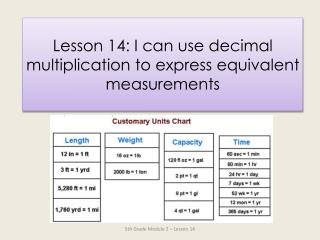DownloadDownload PresentationLesson 14: I can use decimal multiplication to express equivalent measurements

# Lesson 14: I can use decimal multiplication to express equivalent measurements

Download Presentation## Lesson 14: I can use decimal multiplication to express equivalent measurements

- - - - - - - - - - - - - - - - - - - - - - - - - - - E N D - - - - - - - - - - - - - - - - - - - - - - - - - - -
##### Presentation Transcript

1. Lesson 14: I can use decimal multiplication to express equivalent measurements 5th Grade Module 2 – Lesson 14

2. Let’s divide by multiples of 10! Solve the Division Sentence 420 ÷ 10 =____ 42 42 ÷ 2 =___ 21 Solve the Division Sentence Write 420 ÷ 20 as a three-step division sentence taking out the ten. 420 ÷ 20 =___ 420 ÷ 10 ÷ 2 = 42 ÷ 2 =___ 21 420 ÷ 20 =____ 21 5th Grade Module 2 – Lesson 14

3. Let’s divide by multiples of 10! Solve the Division Sentence 960 ÷ 10 =____ 96 96 ÷ 3 =___ 32 Solve the Division Sentence Write 960 ÷ 30 as a three-step division sentence taking out the ten. 960 ÷ 30 =___ 960 ÷ 10 ÷ 3 = 96 ÷ 3 =___ 32 960 ÷ 30 =____ 32 5th Grade Module 2 – Lesson 14

4. Let’s divide by multiples of 10! Solve the Division Sentence 680 ÷ 10 =____ 68 68 ÷ 2 =___ 34 Solve the Division Sentence Write 680 ÷ 20 as a three-step division sentence taking out the ten. 680 ÷ 20 =___ 680 ÷ 10 ÷ 2 = 68 ÷ 2 =___ 34 680 ÷ 20 =____ 34 5th Grade Module 2 – Lesson 14

5. Time to Convert Get Your White Board Ready! 1 meter = ______ centimeters 1 m 50 cm = ______ centimeters 100 150 1 m 5 cm = ______ centimeters 2 meters = ______ centimeters 200 105 2 m 30 cm = ______ centimeters 2 m 7 cm = ______ centimeters 230 207 2 m 90 cm = ______ centimeters 4 m 8 cm = ______ centimeters 290 408 5th Grade Module 2 – Lesson 14

6. Time to Convert Get Your White Board Ready! 12 13 1 foot = _____ inches 1 ft 1 in = _____ inches 14 22 1 ft 2 in = _____ inches 1 ft 10 in = _____ inches 24 2 feet= _____ inches 30 2ft 6 in = _____ inches 5th Grade Module 2 – Lesson 14

7. Try Some More! 34 25 2ft 10 in = _____ inches 2 ft 1 in = _____ inches 36 46 3 feet = _____ inches 3ft 10 in = _____ inches 37 3 ft 1 in= _____ inches 40 3 ft 4 in = _____ inches 5th Grade Module 2 – Lesson 14

8. Time to Convert Get Your White Board Ready! 12 inches = ______ foot 13 inches= ___ ft ___ in 1 1 1 14 inches= ___ ft ___ in 22 inches= ____ft _____ in 2 1 1 10 24 inches= ____ft _____ in 34 inches= ____ft _____ in 2 0 2 10 25 inches= ____ft _____ in 46 inches= ____ft _____ in 2 1 3 10 5th Grade Module 2 – Lesson 14

9. Application Problem #1 36 120 1,332 Click to Reveal Solution 5th Grade Module 2 – Lesson 14

10. Application Problem #2 See Lesson Plan for a Hands-On Activity 5th Grade Module 2 – Lesson 14

11. Concept Development Problem 1 7.43 kilometers = ______ meters Today we will be converting units with decimals. Yesterday we converted from large units to smaller units using multiplication. How will we solve this one? Turn & Talk Does our solution make sense? We will multiply since kilometers is larger than meters. What will we multiply 7.43 by ? 1,000 Solve this problem on your white board. 7.43 x 1000 = ____ meters 7430 5th Grade Module 2 – Lesson 14

12. Concept Development Problem 2 1.8 miles = ______ yards 1 Mile = 1,760 Yards Which part of your reference sheet will help you solve this problem? Tell your partner. What operation would we use to solve this problem? Hmmm….Big to Small… Multiply or Divide? • 1760 1760 • x 1.8x10x 18 • *Solve using ink tool Write the equation that would solve this problem. 1.8 x 1760 = ______ Solve this conversion. Think about how you can adjust 1.8 to a more friendly whole number to help you multiply. 5th Grade Module 2 – Lesson 14

13. Problem 3 0.83 kilograms = ______ grams = ______ milligrams Use Your reference Sheet to help you solve this problem independently. Volunteer to Share their Solution 5th Grade Module 2 – Lesson 14

14. Get Ready to Complete theProblem Set on Your Own! Complete Pages 2.D.21 & 2.D.22 You will have 20 minutes to work. Try your Best! 5th Grade Module 2 – Lesson 14

15. LET’S Debrief • What do you notice about Problem 1(g)? How is it different than the other conversions? How did this difference affect how you solved? • Look back at the chart and your work in Problem 3(d). What do you think about the size of the Statue of Liberty? How does the question help you realize how big she is? 5th Grade Module 2 – Lesson 14

16. EXIT TICKET Page 2.D.23 5th Grade Module 2 – Lesson 14